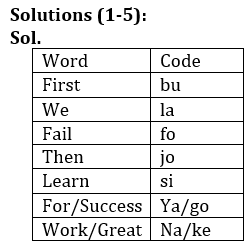Latest Banking jobs   »

# Reasoning Ability Quiz For IDBI AM/ Bank of India PO 2023 -20th March

Directions (1-5): Study the following information to answer the given question.
For a certain code,
“First we fail” is written as “fo la bu”,
“Then we learn” is written as “jo la si ”,
“First learn for success” is written as “ya si bu go”
“Work for great success” is written as “na ya go ke”.

Q1. What does ‘ke’ stand for?
(a) work
(b) great
(c) For
(d) Success`
(e) Either (a) or (b)

Q2. What is the code for ‘ya’?
(a) work
(b) great
(c) For
(d) Success
(e) Either (c) or (d)

Q3. Which of the following may represents ‘We work for success’?
(a) ya la ke si
(b) jo si go la
(c) la go ke ya
(d) bu ya ke go
(e) none of these

Q4. What does ‘jo’ stand for?
(a) Then
(b) Great
(c) For
(d) Success
(e) Either (a) or (b)

Q5. Which of the following may represent ‘We Success’?
(a) la ya
(b) la go
(c) ya go
(d) go jo
(e) Either (a) or (b)

Directions (6-10): Study the following arrangement carefully and answer the questions given below.

V 7 W \$ Y 2 B E 6 # 9 M Q 3 % T @ R © U K 5 D ∞ H 8 G & Z N π 4 P

Q6. Which of the following is the seventh to the left of the fifteenth from the left end of the given arrangement?
(a) #
(b) E
(c) 2
(d) \$
(e) None of these

Q7. How many such numbers are there in the given arrangement, each of which is immediately preceded by a consonant and immediately followed by a symbol?
(a) Three
(b) Two
(c) None
(d) One
(e) More than three

Q8. How many such symbols are there in the given arrangement, each of which is immediately followed and immediately preceded by a number?
(a) None
(b) One
(c) Two
(d) Three
(e) More than three

Q9. Which of the following is the seventh to the left of the tenth from the right end of the given arrangement?
(b) R
(c) @
(d) T
(e) None of these

Q10. What should come in the place of question mark (?) in the following series based on the above arrangement?
7\$2 #M3 RU5
(a) ZN4
(b) 8&N
(c) 8GZ
(d) πZG
(e) None of these

Directions (11-15): Following questions are based on the five three-digit numbers given below.

792 813 541 462 692

Q11. If the positions of the second and the third digits of each of the numbers are interchanged then, how many even numbers will be formed?
(a) None
(b) One
(c) Four
(d) Three
(e) Two

Q12. If all the digits in each of the numbers are arranged in increasing order within the number, then, which of the following number will become the second lowest in the new arrangement of numbers?
(a) 792
(b) 462
(c) 813
(d) 692
(e) None of these

Q13. If one is added to the second digit of each of the numbers and one is subtracted to the third digit of each number then, how many numbers thus formed will be divisible by three in new arrangement?
(a) Two
(b) One
(c) None
(d) Three
(e) Four

Q14. What will be the difference when Second digit of the 3rd lowest number is multiplied with the third digit of the highest number and third digit of the 2nd highest number is multiplied with the second digit of the lowest number?
(a) 22
(b) 27
(c) 13
(d)15
(e) None of these

Q15. If all the numbers are arranged in ascending order from left to right then, which of the following will be the sum of all the three digits of the number which is 2nd from the right in the new arrangement?
(a) 17
(b) 16
(c) 18
(d) 15
(e) None of these

SolutionsSolutions (6-10):
S6. Ans.(b)
S7. Ans.(d)
Sol. Q3%
S8. Ans.(b)
Sol. 6#9
S9. Ans.(c)
S10. Ans.(b)

Solutions (11-15):
S11.Ans(e)
S12.Ans(e)
S13.Ans(d)
S14.Ans(d)
S15.Ans(c)## FAQs

### What is the selection process of the IDBI AM?

The selection process of IDBI AM is Online exam and Interview.

#### Congratulations!Union Budget 2023-24: Free PDF